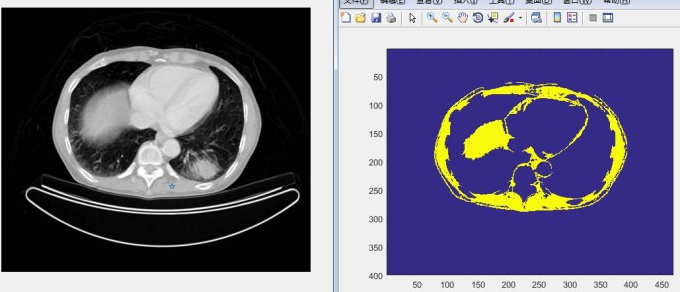• matlab区域分割
2021-05-06 09:02:08

实验十医学图像分割(二)

实验目的：

1.了解图像分割的基本理论和方法；

2.掌握阈值分割的方法和阈值的选择；

3.掌握基于分水岭分割的原理和应用；

实验内容：

1.区域生长法利用图像像素间的相似性进行分割，调用

regiongrow函数对图像weld.tif进行处理，注意参数中S(种

子值)，T(阈值)的选择对分割效果的影响。S=255，T＝

65和S＝255 T＝150和S＝150，T＝65三组值进行处理，

理解在区域生长法的原理。同时对liver.bmp，自己选择合

适的S和T，以较好得分割出肝脏。

代码1：

close all;

figure(1),imshow(f),title('原图');

[g1,NR]=regiongrow(f,255,65);

[g2,NR]=regiongrow(f,255,150);

[g3,NR]=regiongrow(f,150,65);

figure(2),imshow(g1),title('S=255,T=65');

figure(3),imshow(g2),title('S=255,T=150');

figure(4),imshow(g3),title('S=150,T=65');

更多相关内容
• matlab区域分割法代码情报局 ISI 图像采集、刺激呈现和鼠标视觉区域分割的代码。 成像器和刺激器程序 Imager.zip 包含由 Dario Ringach 和 Ian Nauhaus 编写的图像采集文件。 Stimulator.zip 包含用于在数据采集期间...
• matlab区域分割法代码使用 GridCut  进行分割的轻量级 MATLAB 包。 它最小化了表单上给出的函数： Data_term + weight*|boundary length|, 参见 ，了解理论细节。 入门 获取 MATLAB 和兼容的C++编译器：. 使用...
• 对一幅图片将其中间的文字区域定位，并分割出每行 对一幅图片将其中间的文字区域定位，并分割出每行。
• 图像中心定点 进行区域分割，时候用于人脸图像的局部分割图像处理
• 基于MATLAB的图像阈值与区域分割研究.pdfmatlab
• 采用区域生长的方式分割图像，用户可以用鼠标在其中选取一个种子点并按下回车键，之后会出现分割结果。
• 区域生长法分割matlab代码球体成像 项目 6 - 多细胞肿瘤球体的分割 Baptiste Alberti - Tanguy Pace - Titouan Poquillon - Pierrick Ullius 文件的组织 代码文件夹 该文件夹包含所有 Matlab 代码文件和 Ilastik ...
• 基于matlab区域生长的肝影像分割系统，通过区域生长法进行医学影像分隔并结合不同的处理方法进行效果改进，仿真代码亲测可用，有很高的参考价值
• 基于区域生长法的图像分割matlab代码明显的结果 该存储库中的代码是Shih和Cheng撰写的论文“用于彩色图像分割的自动播种区域生长”的MATLAB实现。 该方法包括4个主要部分： 将RGB图像转换为YCbCr颜色空间 自动选种 ...
• matlab基于 K-means 聚类算法的图像区域分割matlab 算法 class 编程语言 Matlab
• 基于区域生长法的图像分割matlab代码 DIPBigHW 下载此代码 命令行下载 git clone https://github.com/githubxiaowei/DIPBigHW.git 或者如图直接点击下载： 数据集下载 数 据 集 下 载 链 接 （ 图 像 + 所 有 标 注 ...
• 基于区域生长法的图像分割matlab代码这些代码在“磁化传递加权EPI有助于原生fMRI空间中皮层深度的确定”一文中使用。 它使用了公开可用的软件包，包括AFNI，SPM，ANT，FreeSurfer和LAYNII。 数据位于 作者：柴玉辉，...
• 1、区域的分离和聚合 步骤： 1）根据条件判断是否将当前图像块进行四分，开始时是整张图像，（进行的是平均四分，所以图像大小需要是2的幂次），然后是小图像块，进行不断划分，知道满足停止条件。 2）对相邻小块...

# 1、区域的分离和聚合

步骤：

1）根据条件判断是否将当前图像块进行四分，开始时是整张图像，（进行的是平均四分，所以图像大小需要是2的幂次），然后是小图像块，进行不断划分，知道满足停止条件。

2）对相邻小块像素进行合并，imreconstruct用于此，知道不能合并

qtdecomp函数是用来分解图像的，（四叉树分解），

Z=qtdecomp(f,@split_test,parameters)，

将f传入到函数split_test中，split这个函数其实就是起到调用predicate函数的作用，predicate函数是判断是否需要分的条件函数。

function v=split_test(B,mindim,fun)
K=size(B,3);%B就是qtdecomp函数传过来的，代表当前size(B,3)返回的是B的层数，就是B是几维的，这里实际上就是有几个B这样大小的图像块
%这句代码的意思是从qtdecomp函数传过来的B，是当前分解成的K块的m*m的图像块，K表示有多少个这样大小的图像块
v(1:K)=false;
for I=1:K
v(I)=false;
continue
end
if flag%如果flag是1，代表需要再分
v(I)=true;%这里就相当于split_test是起一个调用predicate的作用，返回的就是ppredicate的值
end
end
end

function flag=predicate(region)%和图像标准差和均值有关
sd=std2(region);
m=mean2(region);
flag=(sd>22)&(m>5)&(m<255);%参数自己根据实际情况调整
end

主函数

clc
clear

g=splitmerge(f,8,@predicate);
figure,imshow(g)

splitmerge就是主要实现区域分离和合并的函数

function g=splitmerge(f,mindim,fun)%f是待分割的原图，mindim是定义分解中所允许的最小的块，必须是2的正整数次幂
Q=2^nextpow2(max(size(f)));
[M,N]=size(f);
S=qtdecomp(f,@split_test,mindim,fun);%f传给split_test，qtdecomp divides a square image into four
% equal-sized square blocks, and then tests each block to see if it
% meets some criterion of homogeneity. If a block meets the criterion,
% it is not divided any further. If it does not meet the criterion,
% it is subdivided again into four blocks, and the test criterion is
% applied to those blocks. This process is repeated iteratively until
% each block meets the criterion. The result can have blocks of several
% different sizes.S是包含四叉树结构的稀疏矩阵，存储的值是块的大小及坐标，以稀疏矩阵形式存储
Lmax=full(max(S(:)));%将以稀疏矩阵存储形式存储的矩阵变换成以普通矩阵（full matrix）形式存储，full，sparse只是存储形式的不同
g=zeros(size(f));
MARKER=zeros(size(f));

for k=1:Lmax
[vals,r,c]=qtgetblk(f,S,k);%vals是一个数组，包含f的四叉树分解中大小为k*k的块的值，是一个k*k*个数的矩阵，
%个数是指S中有多少个这样大小的块，f是被四叉树分的原图像，r，c是对应的左上角块的坐标如2*2块，代表的是左上角开始块的坐标

if ~isempty(vals)
for I=1:length(r)
xlow=r(I);
ylow=c(I);
xhigh=xlow+k-1;
yhigh=ylow+k-1;
region=f(xlow:xhigh,ylow:yhigh);%找到对应的区域
flag=feval(fun,region);%evaluates the function handle, fhandle,using arguments x1 through xn.执行函数fun，region是参数
if flag%如果返回的是1，则进行标记
g(xlow:xhigh,ylow:yhigh)=1;%然后将对应的区域置1
MARKER(xlow,ylow)=1;%MARKER矩阵对应的左上角坐标置1
end
end
end
end

g=bwlabel(imreconstruct(MARKER,g));%imreconstruct默认2D图像8连通，这个函数就是起合的作用
g=g(1:M,1:N);%返回原图像的大小
end

展开全文图像处理 matlab
• MATLAB编写的基于区域生长方法实现的图像分割
• 将彩色图像通过HSI通道分离，在单通道内区域分割，再将数据重叠在一起，有效的分割目标与背景
• 基于区域融合的半监督的图像分割算法。首先在背景和前景手动设置初始分割标记，在迭代过程中不断通过区域融合操作获得最大相似度的区域，从而实现目标分割
• MATLAB实现的图像分割程序，通过读入一副图片，进行二值化和相应的分割算法之后，可以提取到相应的图像块，并且在不同的分割块上进行标注。代码中附带图片，可以直接运行。
• 具体实现时，先在分割区域找一个种子像素作为生长的起点，再将种子像素周围邻域中与种子像素有相同或相似性质的像素（根据某种事先确定的生长准则确定）合并到种子像素所在区域中，然后将这些新增的像素点作为新的...

区域生长的基本思想是将具有相似性质的像素集合起来构成一个区域，实质是将具有相似特性的像素元素连接成区域。具体实现时，先在分割的区域找一个种子像素作为生长的起点，再将种子像素周围邻域中与种子像素有相同或相似性质的像素（根据某种事先确定的生长准则确定）合并到种子像素所在区域中，然后将这些新增的像素点作为新的生长点重复上面的过程。

三个问题：

1. 选择或确定一组正确代表所需区域的种子像素；

2. 确定生长过程中能将相邻像素包括进来的准则；

3. 生长过程停止的条件或准则。

• 测试图片•  源码
function J = My_RegionGrowing(I, init_pos, reg_maxdist)
% 区域生长法提取目标区域：比较新像素所在区域平均灰度值与各领域像素的灰度值
% 输入：
%    I : 二维数组，数值表示灰度值，0~255
%    init_pos: 指定的种子点坐标
%    reg_maxdist : 阈值，默认值为20
% 输出：
%   J : 感兴趣区域
% 示例：
%{
I = rgb2gray(img);
I = double(I);
x = 271;
y = 259;
J = My_RegionGrowing(I, [x, y], 20);
imshow(img)
hold on
plot(y, x, 'p')
hold off
figure
imagesc(J)
%}
[row, col] = size(I);               % 输入图像的维数
J = zeros(row, col);               % 输出
x0 = init_pos(1);                   % 初始点
y0 = init_pos(2);
reg_mean = I(x0, y0);       % 生长起始点灰度值
J(x0, y0) = 1;                    % 生长起始点设置为白色
reg_sum = reg_mean;                     % 符合生长条件的灰度值总和
reg_num = 1;                                  % 符合生长条件的点的个数
count = 1;                                       % 每次判断周围八个点中符合条件的数目
reg_choose = zeros(row*col, 2);      % 记录已选择点的坐标
reg_choose(reg_num, :) = init_pos;
num = 1;               % 第一个点
while count > 0
s_temp = 0;                  % 周围八个点中符合条件的点的灰度值总和
count = 0;
for k = 1 : num      % 对新增的每个点遍历，避免重复
i = reg_choose(reg_num - num + k, 1);
j = reg_choose(reg_num - num +k, 2);
if J(i, j) == 1 && i > 1 && i < row && j > 1 && j < col   % 已确定且不是边界上的点
% 八邻域
for u =  -1 : 1
for v = -1 : 1
% 未处理且满足生长条件的点
if J(i + u, j + v) == 0 && abs(I(i + u, j + v) - reg_mean) <= reg_maxdist
J(i + u, j + v) = 1;           % 对应点设置为白色
count = count + 1;
reg_choose(reg_num + count, :) = [i + u, j + v];
s_temp = s_temp + I(i + u, j + v);   % 灰度值存入s_temp中
end
end
end
end
end
num = count;                                      % 新增的点
reg_num = reg_num + count;              % 区域内总点数
reg_sum = reg_sum + s_temp;            % 区域内总灰度值
reg_mean = reg_sum / reg_num;         % 区域灰度平均值
end

展开全文图像分割 Matlab图像处理
• 阈值分割后显示分割区域MATLAB代码紧身衣 小脑椎间盘切除术。 在容器中，管道安装在/opt/cersuit目录中。 Matlab代码在src目录中，入口点是src/cersuit.m 。 在bin可以在没有Matlab许可证的情况下在奇异容器中使用的...
• 实现计算机视觉(Computer Vision)中的Haralick区域增长算法(Haralick Region Growing Algorithm)用于实现图像分割
• 基于区域合并的纹理图像分割—MSRM算法的MATLAB实现.doc
• matlab 基于阈值分割区域生长的医学图像分割3讲matlab 编程语言 Matlab
• 5行行Python代代码码实实现现图图像像分分割割的的步步骤骤详详解解 这篇文章主要介绍了5行Python代码实现图像分割的步骤详解文中通过示例代码介绍的非常详细对大家的学习 或者工作具有 定的参考学习价值需要的朋友...
• 对TM灰度图像进行基于梯度幅度的分水岭变换分割，并标记分割区域边界，Matlab源代码，直接拷贝至Matlab中运行。
• 图像分割是一种重要的图像分析技术。对图像分割的研究一直是图像技术研究中的热点和焦点。医学图像分割是图像分割的一...图像分割能够自动或半自动描绘出医学图像中的解剖结构和其它感兴趣的区域，从而有助于医学诊断。...

# matlab区域分割matlab 订阅